# AlgebraPage 3

#### WATCH ALL SLIDES

Slide 30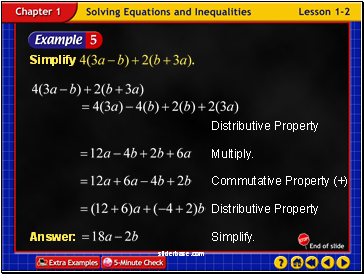Example 2-5a

Slide 31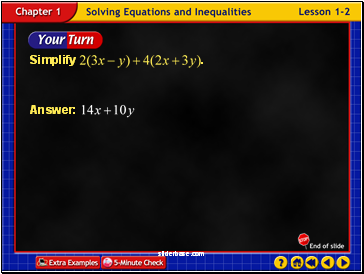Example 2-5b

Slide 32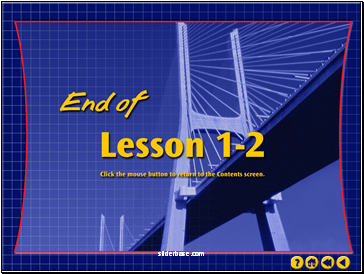End of Lesson 2

Slide 33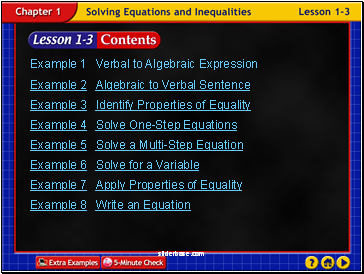Lesson 3 Contents

Example 1 Verbal to Algebraic Expression

Example 2 Algebraic to Verbal Sentence

Example 3 Identify Properties of Equality

Example 4 Solve One-Step Equations

Example 5 Solve a Multi-Step Equation

Example 6 Solve for a Variable

Example 7 Apply Properties of Equality

Example 8 Write an Equation

Slide 34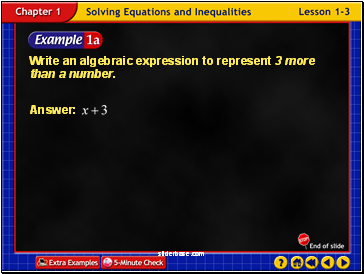Example 3-1a

Write an algebraic expression to represent 3 more than a number.

Slide 35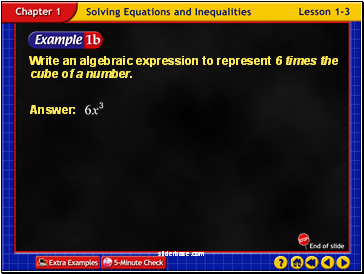Example 3-1b

Write an algebraic expression to represent 6 times the cube of a number.

Slide 36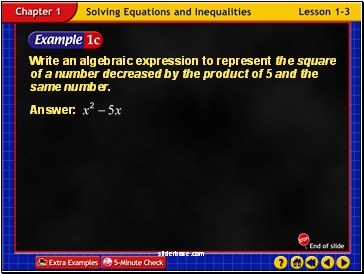Example 3-1c

Write an algebraic expression to represent the square of a number decreased by the product of 5 and the same number.

Slide 37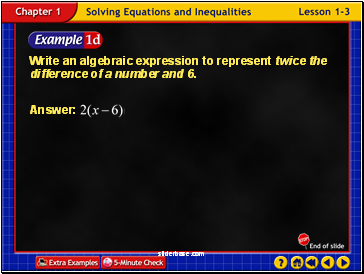Example 3-1d

Write an algebraic expression to represent twice the difference of a number and 6.

Slide 38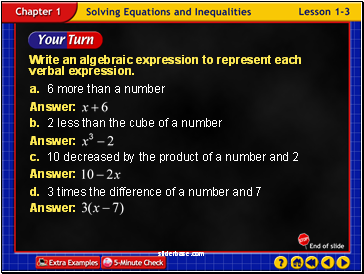Write an algebraic expression to represent each verbal expression.

a. 6 more than a number

b. 2 less than the cube of a number

c. 10 decreased by the product of a number and 2

d. 3 times the difference of a number and 7

Example 3-1e

Slide 39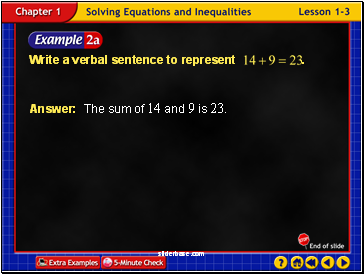Example 3-2a

Answer: The sum of 14 and 9 is 23.

Slide 40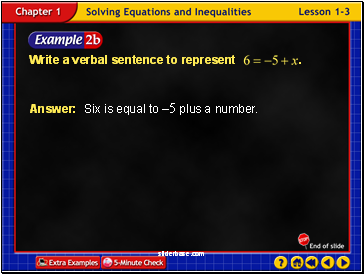Example 3-2b

Answer: Six is equal to –5 plus a number.

Slide 41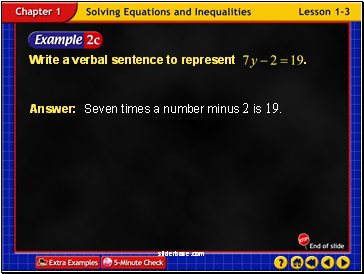Example 3-2c

Answer: Seven times a number minus 2 is 19.

Slide 42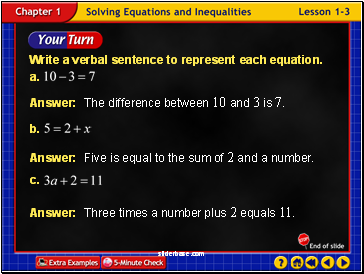Example 3-2d

Answer: The difference between 10 and 3 is 7.

Answer: Three times a number plus 2 equals 11.

Answer: Five is equal to the sum of 2 and a number.

Slide 43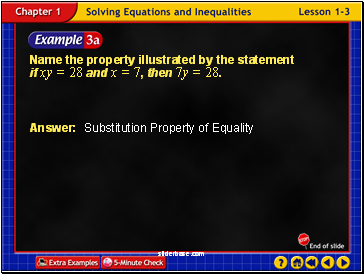Example 3-3a

Name the property illustrated by the statement if xy = 28 and x = 7, then 7y = 28.

Go to page:
1  2  3  4  5  6  7  8